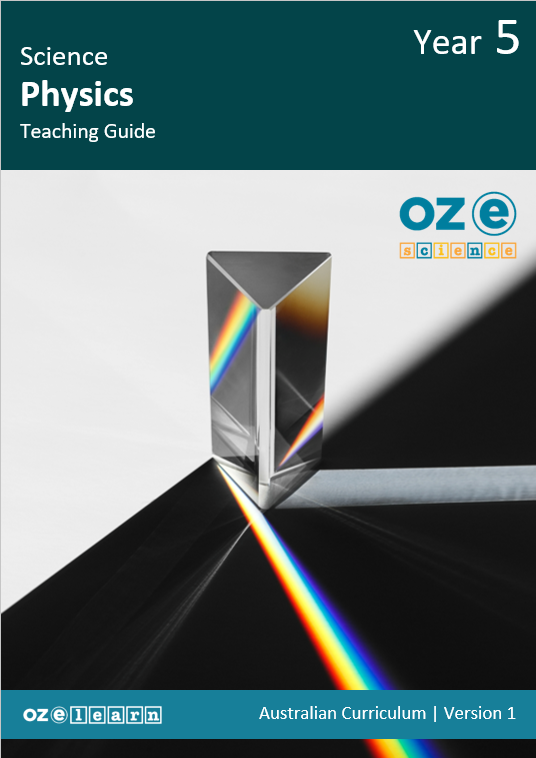# Oz-e-science

Physics - Year 5### Overview

Physics Year 5 is the study of how objects can have an effect on other objects even when they are not in contact with them. It aligns to the Australian Curriculum Science Understanding:
• Light from a source forms shadows and can be absorbed, reflected and refracted (ACSSU080).

No
te: This curriculum program is currently being updated to align with the Australian Curriculum 9.0. Updated versions will be released in early 2024.
Learning Objectives
In lessons 1 to 9, students learn about:
• what a photon is, how they are created in the Sun’s core and how long they take to travel to the surface
• how fast photons can move through space
• how we can see light
• how light can only pass through some materials and not others
• a single ray of light can be bent and split into many different colours and directions
• colour is caused by light
• light is unique because it behaves both like a wave but also like a particle
• applying the Scientific Method to prove that light can move like a particle
• evaluating and communicate your findings to the class.
Success Criteria
• Describe what a photon is.
• Explain how photons are created.
• Explain how photons make their way to the Sun’s surface.
• ͏describe the speed of light.
• Explain how the light we see today can be very old.
• Compare how long it takes light to reach us from different objects.
• ͏Describe how we see light.
• Explain what a reflection is.
• Compare how light reflects from different surfaces.
• Describe the terms transparent, opaque, translucent and absorption.
• Explain how density impacts the absorption of light.
• Compare how light is absorbed by different materials.
• ͏Describe what happens to the path of light when it hits different objects.
• Explain what refraction is.
• ͏Compare how light is bends and splits using different materials.
• ͏Describe how colour is made from light.
• Explain what the light spectrum is.
• Observe how light can be used to create colour.
• ͏Describe how the colour of light changes when it moves towards us or away from us.
• Explain the Doppler effect.
• ͏Observe the Doppler effect using sound.
• ͏Describe how objects are moved because of gravity.
• Explain how light can be bent by very large objects.
• Represent how light bends around a heavy object in space.
• ͏Analyse how scientists first discovered black holes.
• Explain how and why black holes form.
• Represent how a black hole traps light.
• ͏Explain what a laser is.
• Describe how scientists use lasers to map out the universe.
• Represent the mapping of the universe using a small laser.
• ͏Explain what astrophysics is.
• Describe the tools and knowledge used in famous theories about space.
• ͏͏Analyse the famous equation E = mc2.
• Identify the steps in the Scientific Method.
• Experiment using the Scientific Method to explore light.
• Investigate materials to see how much light is let through.
• ͏Explain how waves and particles move.
• Describe how we know light moves like both a wave and a particle.
• ͏͏Analyse the famous light experiment that proves light move like a particle.
• Create a scientific question.
• Create a hypothesis for an experiment.
• Predict the outcome of an experiment.
• Plan an experiment.
• Measure the patterns made by light to determine if it is moving like a wave or a particle.
• Evaluate the results of an experiment.
• Explain the findings using the wave theory of light.
• Communicate findings to the class.
Assessment
Progress Tests
Progress tests are conducted after every second lesson, allowing teachers to monitor student understanding of the concepts taught over the past two lessons and to identify where reteaching is needed. The Teaching Guide contains the testing questions, and the Student Workbook has a section where students write their answers and score themselves.

Structured Research Activity
The Structured Research Activity (SRA) for this unit is: Students to investigate a light school experiment. The SRA takes place over two lessons so students can apply the Science Understanding and Science Inquiry Skills covered in the unit. Teachers use the Guide to Making Judgements, which is included in the Teaching Guide, to mark the SRA.

End-of-Unit Assessment
The last lesson is the end-of-unit assessment which has a variety of question formats (e.g. label the diagram, circle the correct answer) to assess student mastery of content from the unit.

## Recommended Units

\Learnworlds\Codeneurons\Pages\ZoneRenderers\CourseCards